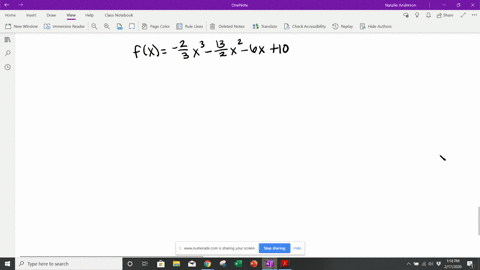Enroll in one of our FREE online STEM summer camps. Space is limited so join now!View Summer Courses### Find the $x$ -value of all points where the funct…

02:44Georgia Southern University

Need more help? Fill out this quick form to get professional live tutoring.

Get live tutoring
Problem 16

Find the $x$ -value of all points where the functions defined as follows have any relative extrema. Find the value(s) of any relative extrema.
$$f(x)=x^{3}+3 x^{2}-24 x+2$$

The value of relative minima will be $-26$ at $x=2$ and value of relative maxima will be of 82 at $x=-4$

## Discussion

You must be signed in to discuss.

## Video Transcript

Okay, so you have dysfunction. That's a hex. Because execute, tell us three X squared twenty four. Thanks. Plus two. All right. Ever asked to find a local extreme the values of those relative extreme. So the first thing we want to do is take the derivative to find the critical points knows their ex values where the function is either at the derivative of the function is either zero r undefined. Those x coordinates are in the domain of functioning as well. So here's the derivative, and this is never going to be under find it just a polynomial. But if we said equal to zero, get a quadratic equation, we could use the quadratic formula, or we could just be hopeful that it factors. And the first thing we notice is that all these coefficients are divisible by three close to about three. So get X squared plus two x st. And if I try to factor this, get it serious? Uh, cause Yeah, good. Here. Zero Nicole's. Hey, Well, see, I need two numbers that multiple. I d give me negative eight and had to give me two. So should be negative for into you spoil this hour. You should get same thing that you do, sir. Critical points are negative for and too right. So now we can draw our number. Land implied thank you for and Teo. And we want to test values of the derivatives to see whether they're positive or negative to see whether the function is increasing or decreasing around these critical points. Uh, *** Negative five to the left of negative for when you pick something Betweennegative twos because zero. Then we can pick three. And I wanna plug these red values into the derivatives. If I plug it into five in seeing it's seventy five minus thirty miles. Twenty four, that's going to be positive. And I really only care about the sign on the door of grease that has a functioning saying at zero again negative talk for easy to see. It's of its negative zero and then three twenty seven points seventeen. I strongly for that's very positive. Can unplugging these into the derivative, right? So now we see the general shape of our Graff. We are increasing up to negative for than decreasing, then increasing after two. So that's kind of our graph Looks like, So we have a relative. Maxima had begged for relative minima, too, and we can find the values of those local maximum Emma so negative four. So the plug negative for back into the function to get the value of the local Maxima here. So we have, uh, it's negative down. So the first term negative for cute that's negative. Sixty four and then plus correct three times sixteen or hate and then minus to the twenty four times negative for hiss. Positive ninety six plus thanks and then us two. Good answer. That's going to be all right. So *** before plus forty eight, that's going to give me a value of negative sixteen plys ninety six since takeaway sixteen ninety six eighty and then and to being a game, too. And then the value of the function hat to value of the minimum to local minima. This can't be ate too cute, plus three times for twelve minus twenty four times. Two. Paint us, too. It's twenty miles forty eight nine twenty eight plus two. Thank you tive twenty six p that value